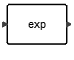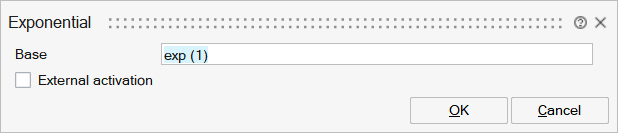# Exponential

This block implements the power function given the base as block parameter: y=a^u where a is the block parameter and u is the input. If a equals exp(1), then the operation corresponds to the exponential function.MathOperations

## Description

The Exponential block implements the power function given the base as block parameter: y=a^u where a is the block parameter and u is the input. If a equals exp(1), then the operation corresponds to the exponential function.

The input u is either a scalar, a vector or a matrix.

The block performs element-wise exponential when input is a matrix. To compute a matrix exponential, use the MatrixExponential block instead.

## ParametersNameLabelDescriptionData TypeValid Values

base

Base

The block implements the power function given this parameter.

Matrix of size 1x1

externalActivation

External activation

Specifies whether the block receives an external activation or inherits its activation through its regular input ports. When External Activation is selected, an additional activation port is added to the block. By default, external activation is not selected.

Number

0
1

## Ports

NameTypeDescriptionIO TypeNumber

Port 1

explicit

output

1

Port 2

explicit

input

1

Port 3

activation

input

externalActivation

NameValueDescription

always active

no

direct-feedthrough

yes

zero-crossing

no

mode

no

continuous-time state

no

discrete-time state

no